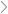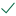Questions & AnswersFinanceRemember that there has to be a negative number in your...

QuestionAnswered step-by-step

# Remember that there has to be a negative number in your...

Remember that there has to be a negative number in your calculations for the formulas to work. If you get an error message on your calculator, go back, and make one of the dollar variables negative......usually the PV factor 😊.

1. Paulo has won the lottery. He is offered a series of payments of \$15,000 per year for 10 years. What is the present value of these payments at an interest/discount rate of 6%?

PV =

FV =

I =

N =

Pmt =

1. Keesha's grandmother died and left a trust for her that pays out \$20,000 per year for 10 years. If Keesha invests the payments at 8%, what will her future value be?

PV =

FV =

I =

N =

Pmt =

1. Bob has been in an accident and the other party's insurance company has offered either a one-time payout of \$15,000, or a series of payments of \$1,100 per year for 15 years.  (al) If Bob knows that the current investment rate of interest is 6%, what is the present value of the series of payments? (b) Which should Bob take based on present values?

PV =

FV =

I =

N =

Pmt =

1. You want to buy a new car that costs \$25,000, and you put 20% down payment on the purchase and finance the remainder. How much would your monthly payments be if you borrow the money for 5 years from a credit union at 4% annually?

Remember: You have to make the term of the loan and the interest rate monthly values to align with the monthly payment you want to compute)

PV =

FV =

I =

N =

Pmt =

1. You want to buy a house that costs \$200,000, you agree with the bank to put 25% down and they will finance the remainder. Because you have a good credit score you have been offered a 20-year loan at 3.6%. What is your monthly payment of principal and interest?

PV =

FV =

I =

N =

Pmt =Solved by verified expert10,000 step-by-step explanations